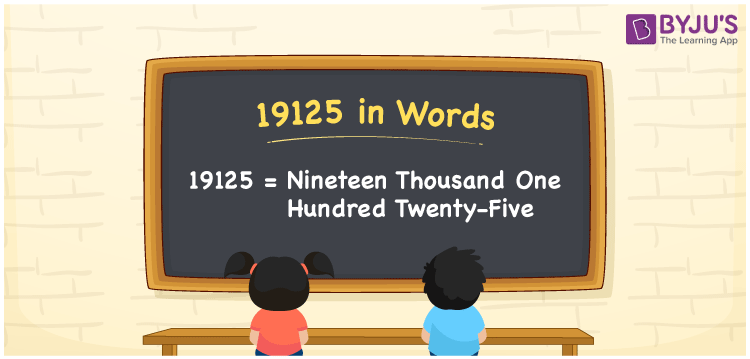# 19125 in Words

19125 in words can be written as Nineteen Thousand One Hundred Twenty-five. The fundamental concepts in Mathematics like counting or count can be learnt efficiently here. If you buy a scooter for Rs. 19125, then you can say that “I bought a scooter for Nineteen Thousand One Hundred Twenty-five Rupees”. To write numbers in words, the English alphabet is used. The numbers in words concept is explained here in a simple way to improve the conceptual knowledge of students. The number 19125 can be read as “Nineteen Thousand One Hundred Twenty-five” in English.

 19125 in words Nineteen Thousand One Hundred Twenty-five Nineteen Thousand One Hundred Twenty-five in Numbers 19125

## 19125 in English Words## How to Write 19125 in Words?

Students can learn about the expanded form and the place value chart of 19125. Five digits are present in the number 19125. With the help of the place value chart given below, students will be able to understand the concepts with ease.

 Ten Thousands Thousands Hundreds Tens Ones 1 9 1 2 5

19125 can be written in expanded form as:

1 x Ten Thousand + 9 x Thousand + 1 × Hundred + 2 × Ten + 5 × One

= 1 x 10000 + 9 x 1000 + 1 × 100 + 2 × 10 + 5 × 1

= 10000 + 9000 + 100 + 20 + 5

= 19125

= Nineteen Thousand One Hundred Twenty-five

Hence, 19125 in words is written as Nineteen Thousand One Hundred Twenty-five.

19125 is a natural number that precedes 19126 and succeeds 19124.

19125 in words – Nineteen Thousand One Hundred Twenty-five

Is 19125 an odd number? – Yes

Is 19125 an even number? – No

Is 19125 a perfect square number? – No

Is 19125 a perfect cube number? – Yes

Is 19125 a prime number? – No

Is 19125 a composite number? – Yes

## Frequently Asked Questions on 19125 in Words

Q1

### How to write 19125 in words?

19125 can be written in words as “Nineteen Thousand One Hundred Twenty-five”.
Q2

### How to write Nineteen Thousand One Hundred Twenty-five in numbers?

Nineteen Thousand One Hundred Twenty-five in numbers can be written as 19125.
Q3

### Is 19125 an odd or even number?

19125 is an odd number as it is not completely divisible by 2.